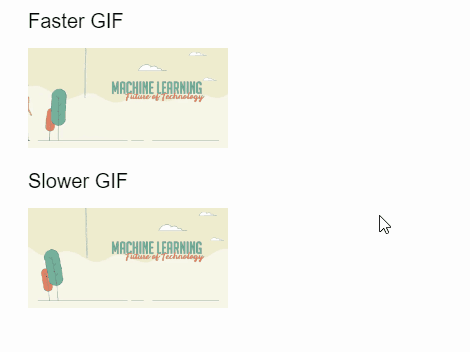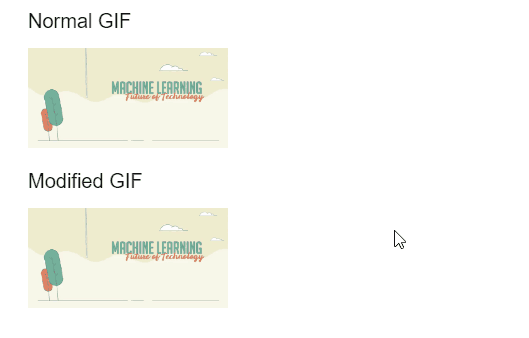Open in App
Not now

# p5.js Image delay() Method

• Last Updated : 30 Sep, 2020

The delay() method of p5.Image in p5.js is used to change the delay between each frame in a GIF animation. This value can be set in milliseconds and a higher value would mean that the GIF would show its next frame after a longer time.

An optional second parameter can be used to specify the index of the frame that needs to have the new delay value set. All the frames would get the new delay value if nothing is specified.

Syntax:

```resize( d, index )
```

Parameters: This function accepts two parameters as mentioned above and described below.

• d: It is a number that specifies the amount to delay between each frame in milliseconds.
• index: It is a number that specifies the index of the frame that has to be modified. It is an optional parameter.

The examples below illustrate the delay() method in p5.js:

Example 1:

## Javascript

 `function` `preload() { ` `    ``faster_gif = loadImage(``"sample-gif.gif"``); ` `    ``slower_gif = loadImage(``"sample-gif.gif"``); ` `} ` ` `  `function` `setup() { ` `    ``createCanvas(500, 300); ` `    ``textSize(20); ` ` `  `    ``text(``'Faster GIF'``, 20, 20); ` `    ``text(``'Slower GIF'``, 20, 180); ` ` `  `    ``// Speed up the GIF with a delay ` `    ``// of 10 milliseconds between ` `    ``// each frame ` `    ``faster_gif.delay(10); ` ` `  `    ``// Slow down the GIF with a delay ` `    ``// of 100 milliseconds between ` `    ``// each frame ` `    ``slower_gif.delay(100); ` `} ` ` `  `function` `draw() { ` `    ``image(faster_gif, 20, 40, 200, 100); ` `    ``image(slower_gif, 20, 200, 200, 100); ` `}`

Output:Example 2:

## Javascript

 `function` `preload() { ` `    ``normal_gif = loadImage(``"sample-gif.gif"``); ` `    ``modified_gif = loadImage(``"sample-gif.gif"``); ` `} ` ` `  `function` `setup() { ` `    ``createCanvas(500, 300); ` `    ``textSize(20); ` ` `  `    ``text(``'Normal GIF'``, 20, 20); ` `    ``text(``'Modified GIF'``, 20, 180); ` ` `  `    ``// Modify the GIF with the delay ` `    ``// applied to the 100th frame ` `    ``modified_gif.delay(4000, 100); ` `} ` ` `  `function` `draw() { ` `    ``image(normal_gif, 20, 40, 200, 100); ` `    ``image(modified_gif, 20, 200, 200, 100); ` `}`

Output:Online editor: https://editor.p5js.org/
Environment Setup: https://www.geeksforgeeks.org/p5-js-soundfile-object-installation-and-methods/
Reference: https://p5js.org/reference/#/p5.Image/delay

My Personal Notes arrow_drop_up
Related Articles